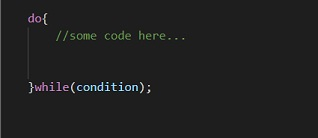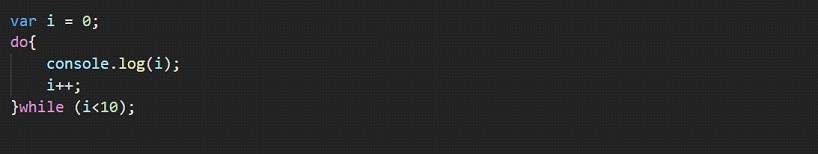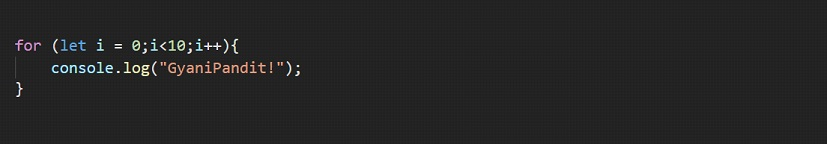# Do While Loop Javascript

Do While Loop Javascript

The do… while loop is another kind of loop. We now know that looping just means doing something repeatedly. So, all we are doing is doing something repeatedly. We have seen that in the while loop Javascript, every time we enter the loop, the condition is checked. Only if the condition is true, do we enter the loop. But here, the thing is quite different. You will observe this when you get to use it.

## Do While Loop Javascript

Here, the syntax is going to be quite different. This is like… do this(run some code), while this(some condition) is true. Ahead, we are going to have a look at what is different in the while, and the do… while loop. There is a slight difference(apart from the syntax, and apart from the extra do keyword).

Here is the syntax of a do… while loop →Now, since this is again a loop, we have the iteration variable, the condition check, and the increment(change) expression here as well. Let’s have an example, which is again similar to previous examples, but this will give us an idea about the do… while loop JavascriptIf we try running the above program, the output is again 0 to 9. Again here as well, the loop will execute while the condition is true.

Here, you can see that we are running some code, while the condition is true.

Well, now let’s come to an important thing, which is the difference between the while and the do-while loop. Well, I am not talking about some obvious differences, like the syntax, and extra do keyword, but there are some real differences here, which we are going to have a look at. But for that, I want you to observe some things here. Like when are we checking the conditions in both the loops, and at least how many times, both the loops are going to execute? And these points are where the differences lie.

Well, the difference between the while and do…while loop is that the while loop checks the condition before execution. If the condition is true, then only the code inside the while loop is supposed to be executed. But with a do-while loop, when the loop starts, it will execute once, then the condition is checked, and then if the condition is true, then only the loop executes again. This means that the do-while loop will execute at least once.

So, we can simply say that in the while loop, we are checking the condition before going in the loop, and in the do-while loop, we are checking the condition afterward. Also, the do-while loop is going to be executed at least once, even if the condition was never true, which is not the case with the while loop.

Do not worry at all, if you did not get the whole concept. All we need to do is practice, practice, and practice. You can try some different programs, which involve the concept of looping, and try to observe and analyze the output as well.

JUST UNDERSTAND THAT WE ARE EXECUTING SOMETHING REPEATEDLY (FOR MANY TIMES), AND SO WE ARE USING THE LOOPS SO THAT WE DO NOT REQUIRE TO WRITE THE WHOLE CODE FOR SO MANY TIMES.

Before we move on to another concept, let’s have another program, where we are going to do a super simple thing, of printing

! to the console, 10 times. As of now, I think you would be able to do this, and if not, you can at least refer to the below program, and give it a try –If we have a look at the output, we can see GyaniPandit on the console 10 times(0 to 9).

You can try doing the same things with the while and the do-while loops.

So, this was much about the different loops, like the for loop, the while loop, and the do-while loop. You can try different examples which involve looping, so as to get better at the concept. Now, we are going to go ahead, and take a look at some interesting keywords, like the break and continue.